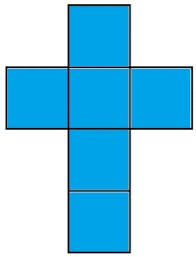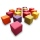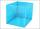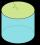# Sum of the edges

The sum of the lengths of all edges of the cube is 72 cm. How many cm2 of colored paper are we going to use for sticking?

S =  216 cm2

### Step-by-step explanation:Did you find an error or inaccuracy? Feel free to write us. Thank you!#### You need to know the following knowledge to solve this word math problem:

We encourage you to watch this tutorial video on this math problem:

## Related math problems and questions:

• Cube edgesThe sum of the lengths of the cube edges is 42 cm. Calculate the surface of the cube.
• Edges or sidesCalculate the cube volume, if the sum of the lengths of all sides is 276 cm.
• Cube 7Calculate the volume of a cube, whose sum of the lengths of all edges is 276 cm.
• Colored paperCan a 4 cm, 3 cm and 2 cm block be glued with a colored quarter of paper measuring 7 cm and 8 cm?
• Two wallsCalculate the surface area of a cube in m2 if you know that the area of its two walls is 72 dm2.
• Cuboid surfaceDetermine surface area of cuboid if its volume is 52.8 cm cubic and length of the two edges are 2 cm and 6 cm.
• Cube 5The surface of the cube is 15.36 dm2. How will change the surface area of this cube if the length of the edges is reduced by 2 cm?
• Cube edgeDetermine the edges of the cube when the surface is equal to 37.5 cm square.
• Cube 1-2-3Calculate the volume and surface area of the cube ABCDEFGH if: a) /AB/ = 4 cm b) perimeter of wall ABCD is 22 cm c) the sum of the lengths of all edges of the cube is 30 cm.
• CubeThe sum of all cube edges is 30cm. Find the surface area of the cube.
• Surface of cuboidFind the surface of the cuboid if its volume is 52.8 cm3 and the length of its two edges is 2 cm and 6 cm.
• Cube 5The content area of one cube wall is 32 square centimeters. Determine the length of its edges, its surface and volume.
• PrismThree cubes are glued into a prism. The sum of the lengths of all its edges is 115 cm. What is the length of one edge of the original cube?
• CubeThe sum of lengths of cube edges is 57 cm. What is its surface and volume?
• The cubeThe cube has a surface of 600 cm2. What is its volume?
• Cube V2SThe volume of the cube is 27 dm cubic. Calculate the surface of the cube.
• Curved surface area CSAA cylinder 5cm high has a base radius(7/2) cm. Calculate the curved surface area.